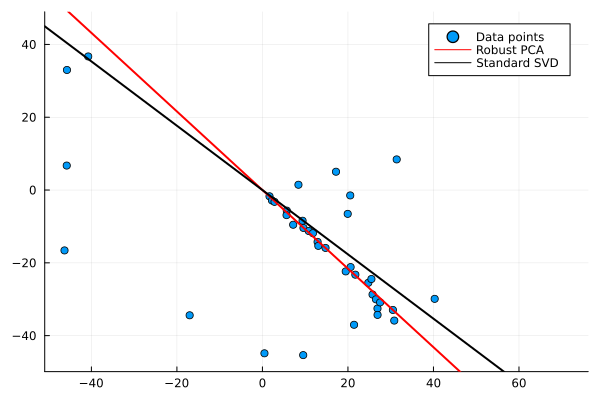# Computing a Robust PCA

For a given matrix $X ∈ ℝ^{p×n}$ whose columns represent points in $ℝ^p$, a matrix $U ∈ ℝ^{p×d}$ is computed for a given dimension $d < n$: $U$ represents an ONB of $ℝ^{p× d}$ such that the column space of $U$ approximates the points (columns of $X$) $X_i$ as well as possible.

We compute $U$ as a minimizer over the Grassmann manifold of the cost function:

$$\begin{split} f(U) & = \frac{1}{n}\sum_{i=1}^{n}{\operatorname{dist}(X_i, \operatorname{span}(U))}\\ & = \frac{1}{n} \sum_{i=1}^{n}\lVert UU^TX_i - X_i\rVert \end{split}$$

The output cost represents the average distance achieved with the returned $U$, an orthonormal basis (or a point on the Stiefel manifold) representing the subspace (a point on the Grassmann manifold). Notice that norms are not squared, so we have a robust cost function. This means that $f$ is nonsmooth, therefore we regularize with a pseudo-Huber loss function of smoothing parameter $ϵ$.

$$f_ϵ(U) = \frac{1}{n} \sum_{i=1}^n{ℓ_ϵ(\lVert UU^{\mathrm{T}}X_i - X_i\rVert)},$$

Where $ℓ_ϵ(x) = \sqrt{x^2 + ϵ^2} - ϵ$.

The smoothing parameter is iteratively reduced (with warm starts).

First, we generate random data for illustration purposes:

using Random
begin
samples = 40
outliers = 15
Random.seed!(42)
X = randn(2, 1) * [1:samples;]' + 0.05 * randn(2, samples) .* [1:samples 1:samples]'
# Outliers:
P = shuffle(1:size(X, 2))'
X[:, P[1:outliers]] = 30 * randn(2, outliers)
d = 1
end;

We use the Manopt toolbox to optimize the regularized cost function over the Grassmann manifold.

To do this, we first need to define the problem structure.

using Manifolds
p, n = size(X);
M = Grassmann(p, d);

For the initial matrix $U_0$ we use classical PCA via singular value decomposition. Thus, we use the first $d$ left singular vectors.

Then, we compute an optimum of the cost function over the Grassmann manifold. We use a trust-region method which is implemented in Manopt.jl.

For this, the objective function robustpca_cost and the Riemannian gradient grad_pca_cost must first be implemented as functions. Since we Huber regularize them, the functions also have the ϵ as a parameter. To obtain the Riemannian gradient we first compute the Euclidian gradient. Afterwards it is projected onto the tangent space by using the orthogonal projection $I-U*U^T$.

The trust-region method also requires the Hessian Matrix. By using ApproxHessianFiniteDifference we get an approximation of the Hessian Matrix.

We run the procedure several times, where the smoothing parameter $ϵ$ is reduced iteratively.

using LinearAlgebra, Manopt, Statistics
begin
ϵ = 1.0
iterations = 6
reduction = 0.5
end;
U, S, V = svd(X);
U0 = U[:, 1:d];
U0
2×1 Matrix{Float64}:
-0.7494248652139397
0.6620893983436593
robustpca_cost(M, U0, 0.0)
10.218786443576352
function grad_pca_cost(M, U, ϵ)
UtX = U' * X
vecs = U * UtX - X
sqnrms = sum(vecs .^ 2; dims=1)

# Compute the Euclidean gradient
G = zeros(p, d)
for i in 1:n
G = G + (1 / (sqrt(sqnrms[i] + ϵ^2))) * vecs[:, i] * UtX[:, i]'
end
G = 1 / n * G
# Convert to Riemannian gradient
return (I - U * U') * G
end
grad_pca_cost (generic function with 1 method)
function robustpca_cost(M, U, ϵ)
vecs = U * U' * X - X
sqnrms = sum(vecs .^ 2; dims=1)
dim = size(sqnrms)
vals = sqrt.(sqnrms + ϵ^2 * ones(dim)) - ϵ * ones(dim)
return mean(vals)
end
robustpca_cost (generic function with 1 method)
begin
Ui = copy(M, U0)
ϵi = ϵ
for i in 1:iterations
global Ui = trust_regions(
M,
(M, p) -> robustpca_cost(M, p, ϵi),
(M, p) -> grad_pca_cost(M, p, ϵi),
ApproxHessianFiniteDifference(
M,
Ui,
(M, p) -> grad_pca_cost(M, p, ϵi);
vector_transport_method=ProjectionTransport(),
retraction_method=PolarRetraction(),
),
Ui;
(project!)=project!,
)
global ϵi = ϵi * reduction
end
end;
Ui
2×1 Matrix{Float64}:
-0.6795640993446082
0.7336161359198359
robustpca_cost(M, Ui, 0.0)
9.412961993577344

Finally, the results are presented graphically. The data points are visualized in a scatter plot. The result of the robust PCA and (for comparison) the standard SVD solution are plotted as straight lines.

using Plots
fig = plot(X[1, :], X[2, :]; seriestype=:scatter, label="Data points");
plot!(
fig,
Ui * [-1, 1] * 100,
Ui * [-1, 1] * 100;
linecolor=:red,
linewidth=2,
label="Robust PCA",
);
plot!(
fig,
U0 * [-1, 1] * 100,
U0 * [-1, 1] * 100;
xlims=1.1 * [minimum(X[1, :]), maximum(X[1, :])],
ylims=1.1 * [minimum(X[2, :]), maximum(X[2, :])],
linewidth=2,
linecolor=:black,
label="Standard SVD",
)